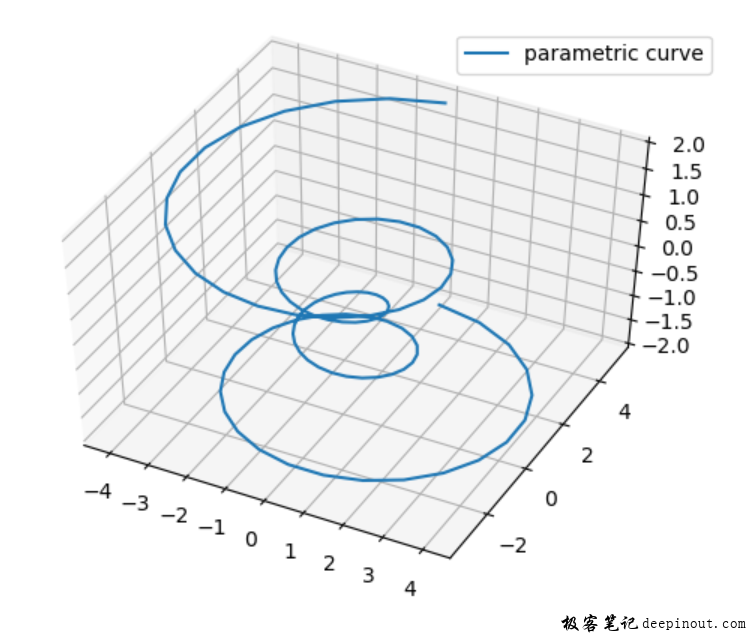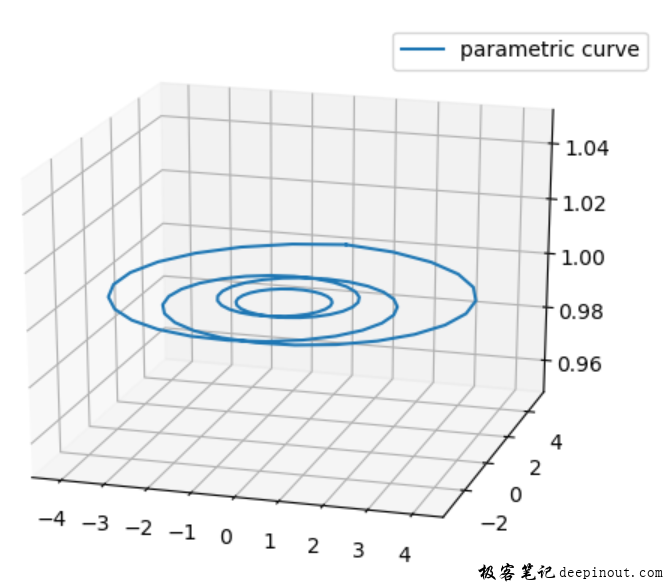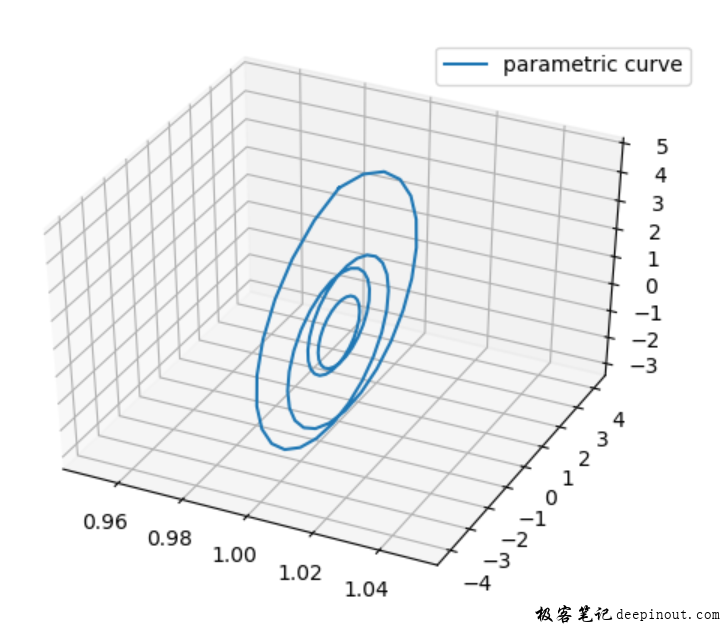# Matplotlib 绘制3D图像theta = np.linspace(-4 * np.pi, 4 * np.pi, 100)
z = np.linspace(-2, 2, 100)
r = z**2 + 1
x = r * np.sin(theta)
y = r * np.cos(theta)

Axes3D.plot(self, xs, ys, *args, zdir='z', **kwargs)

• xs：X轴的坐标数组，一维数组。

• ys：Y轴的坐标数组，一维数组。

• zs：Z轴的坐标值，可以是一个点，也可以是一维数组。

• zdir：可选值：{‘x’, ‘y’, ‘z’}，默认：’z’，当绘制二维数据时，选择那一个作为Z方向。

• **kwargs：它的取值与matplotlib.axes.Axes.plot是一样的。

import numpy as np

from matplotlib import pyplot as plt
from mpl_toolkits import mplot3d

plt.rcParams['legend.fontsize'] = 10
fig = plt.figure()
ax = fig.add_subplot(1,1,1, projection = "3d")

#
theta = np.linspace(-4 * np.pi, 4 * np.pi, 100)
z = np.linspace(-2, 2, 100)
r = z**2 + 1
x = r * np.sin(theta)
y = r * np.cos(theta)

ax.plot(x, y, z, label='parametric curve')
ax.legend()

plt.show()

ax.plot(x, y, 1.0, label='parametric curve')ax.plot(x, y, 1.0, label='parametric curve', zdir = 'x')import numpy as np
from matplotlib import pyplot as plt
from mpl_toolkits import mplot3d

plt.rcParams['legend.fontsize'] = 10

fig = plt.figure()
ax = fig.add_subplot(1,1,1, projection = "3d")

#
theta = np.linspace(-4 * np.pi, 4 * np.pi, 100)
z = np.linspace(-2, 2, 100)
r = z**2 + 1
x = r * np.sin(theta)
y = r * np.cos(theta)

ax.plot(x, y, 1.0, label='parametric curve', zdir = 'x')
ax.legend()

plt.show()

• 微信订阅
• 回顶